Courses

# Introduction To Three Phase Transformer Electrical Engineering (EE) Notes | EduRev

## Electrical Engineering (EE) : Introduction To Three Phase Transformer Electrical Engineering (EE) Notes | EduRev

The document Introduction To Three Phase Transformer Electrical Engineering (EE) Notes | EduRev is a part of the Electrical Engineering (EE) Course Electrical Machines for Electrical Engg..
All you need of Electrical Engineering (EE) at this link: Electrical Engineering (EE)

Introduction to Three Phase Transformer
So far we have discussed the analysis and performance of single phase transformers. However, majority of the transmission network and power distribution network is 3 phase. Therefore, for to be useful, one should ideally consider 3 phase transformer, which we will introduce today.

(Refer Slide Time: 01:32)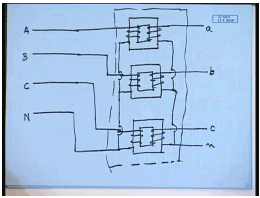Now, that should not be a problem if one has a 3 phase 4 wire incoming line. One can use three single phase transformers of the usual construction that we have been familiar with and connect them to three input lines in this manner – A, B, C and neutral. Similarly, the secondary’s can also be connected in a similar manner and get a 3 phase, four wire output, which is stepped up or step down. So, this three single phase transformer together will constitute a 3 phase transformer. This is very much possible. And in many cases, this is also done. But, something more can be done. Normally, the input voltages will be balanced; that is, V A N, V B N and V C N form a balanced 3 phase system.

(Refer Slide Time: 04:09)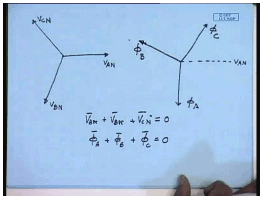This is V A N; this is V B N; this is V C N. We know that, the flux produced – the magnetizing flux produced in each of this transformer lags the corresponding applied voltage by 90 degree. So, let us call them phi A, phi B and phi C. So, phi A, phi B, phi C are also balanced 3 phase phasers. And we can draw them maintaining same phase relationship. So, phi A will lag phi A N by 90 degree; this will be phi A. Phi B will lag phase by 90 degree. And phi C will lag V C N by 90 degree. Just as V A N phasers V A N – V B N plus V C N equal to 0; similarly, from geometry, phi A plus phi B plus phi C is also equal to 0.

Now, this has some implication; which means the sum of these phaser sum of these three fluxes are identically 0. Hence, instead of putting this secondary’s on this arm, if we put the secondary’s on the same arm as the primary and merge the three of the remaining arms, the total flux flowing through that arm – that common arm will be 0. And that total… That common arm can be completely eliminated. Hence, we will arrive at a structure, which will look like this.

(Refer Slide Time: 07:02)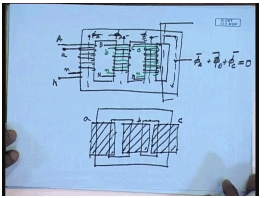This is the arm for A phase – both primary and secondary. And this merged arm… Still the flux produced… This will be phi A; this will be phi B and this will be phi C. And the sum of this flux, which is expected to flow through this merged return path – this will be phi A plus phi B plus phi C. And but this is identically equal to 0. Therefore, this arm is not necessary.

Hence, the simple structure; it becomes something like this with three windings – both primary and secondary. Here three arms carrying respectively 3 phase windings. This is for a phase; this is for b phase; this is for c phase. So, this keeps a 3 phase transformer as a single unit. Therefore, we saw that, we can connect three single phase transformers separately to form a 3 phase transformer. Or, we can build a 3 phase transformer as a single unit. There are advantages and disadvantages of both the approaches, which we will find it; we will see shortly.

(Refer Slide Time: 11:24)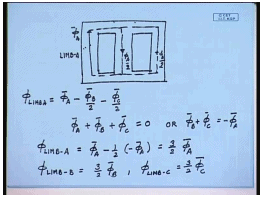Before that, let us look at the core structure a little more carefully. The core structure that we had considered so far looks somewhat like this. Ideally if we assume the core to have infinite permeability; then this yoke section will have zero reluctance; and any flux let us say produced phi A will divide equally here as phi A by 2 and here through this arm as another phi A by 2; similarly, for the flux produced by other phases. Therefore, what will be the total flux flowing through each of these limbs.

So, let us say for the first limb, it will be phi A in the upward direction minus phi B by 2 in the downward direction minus phi C by 2 in the downward direction. This is the net flux flowing through limb 1 or limb-A. But, we have seen phi A plus phi B plus phi C equal to 0 or phi B plus phi C equal to minus phi A. Hence, phi limb-A will be phi A minus half of minus phi A equal to 3 by 2 phi A. Similar exercise can be done for the other limbs. And we will find that phi limb-B will be equal to 3 by 2 phi B and phi limbC will be equal to 3 by 2 phi C.

Now, this is an important conclusion – important observation. We find that, the flux flowing through the limbs are in fact, in phase with the flux produced by the winding on that limb even when there is mutual coupling between the fluxes. Therefore, the operation of the coils on individual limbs will be independent of each other as long as this relationship, that is, the balanced voltage relationship is maintained. Hence, as far as analysis of a 3 phase transformer goes, each phase can be treated separately at least with balanced applied voltage and in steady state. This makes the analysis of 3 phase transformer very simple. They can be balanced; they can be performed just like single phase transformer. Assume if we can find out what is the applied voltage across each winding.

Now, this comment should be taken with a caveat, because we have assumed the iron to have infinite permeability; however, in actual practice, the iron will not have infinite permeability. In fact, since the transformers are operated very close to their knee point, there will be some amount of reluctance to flux path in the yoke. Therefore, the diffusion of the flux in an actual transformer may not be exactly phi by 2 and phi by 2; there will be more flux flowing through the central limb in this case than the farther limb, because this reluctance is higher than this reluctance path.

So, the diffusion will not be exactly phi A by 2 and phi A by 2, except for the middle arm, where the diffusion will possibly be exactly phi B by 2 and phi B by 2. But, for the phase A and phase C, the diffusion will not be exact. Hence, this in a practical transformer, it will not be completely… – each phase will not be completely decoupled, but there will be some amount of coupling; but usually that is negligible. The coupling can be made a little better if we use a different type of core. Incidentally, this is the core type transformer. We call it a 3-limbed transformer core. More regarding construction of 3 phase transformer we will see in a later lecture.

(Refer Slide Time: 17:43)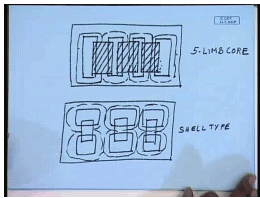But, to continue with this, this is a 3-limbed core, which is most commonly used. However, for very large transformer, sometimes a 5-limbed core is also used. The windings are placed on the inner limbs; while these two limbs do not carry any winding. In this case, we can easily see that, the magnetic circuit for each of the phases are more symmetric than the previous case. And hence, the assumption of independent operation is more valid in this case. But, as we can easily find out that, this core is much larger than the core that we have discussed before; hence, it is justified only for very large rated transformer. This is called 3-limb core type. This is 5-limbed core. There is another construction of the core, which is called the shell type construction; but this central section holds the winding. This also gives for each phase an independent non-interacting flux path.

Here also, the three phases can be treated separately. This is the shell type 3 phase transformer. So, although, 3 phase transformer are most commonly found with 3-limbed core; some very large transformers are also built with 5-limbed core or a shell type core, where the magnetic circuit of each phases are either symmetric or they are independent. But, as you can see, at least for the shell type core, this is little more than three single phase transformers connected together. So, this does not offer the advantages of 3- limbed core; it is seldom used.

Now, let us look at what are the advantages of using a 3 phase transformer built as a single unit and three single phase transformers. It is the common experience is that, if you build a 3 phase transformer as a single unit, then the volume, cost everything are lower compared to if you build the same transformer with three single phase unit. That is a big advantage. However, there are certain advantages of building a 3 phase transformer with three single units.

For example, for maintenance and reliability purpose, it will be sufficient to keep one spare single phase transformer, so that if there is a fault in one of the transformers, the spare single phase transformer can replace the faulty transformer; and the transformer can keep supplying power. However, for a transformer, which is built as a single unit in order to provide redundancy, a full 3 phase transformer is to be kept as standby, which is a more expensive proposition.

In certain applications, such as in mines, this becomes very important, because the space is restricted; therefore, it is convenient to keep a single phase transformer as a spare. And that is why, in mining application, a single phase transformer is preferred. There are several other advantages as we will shortly see; that a single phase transformer even when one of the transformers developed a fault and is consequently removed, the remaining transformer can keep supplying at least a part of the power, which is not possible with a 3 phase transformer. Also, the maintenance and repair of 3 phase transformer are somewhat more difficult. We have discussed how the core of a 3 phase transformer looks like.

(Refer Slide Time: 24:51)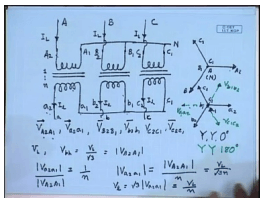Now, let us look at how we can connect the windings of a 3 phase transformer. We have already mentioned that, as far as the magnetic circuit is concerned, the windings operate almost independent of each other. So, let us schematically represent the transformer windings. As you can see, even in the simplest of the 3 phase transformer, whether built with a 3 single phase transformer or built as a single unit, there are six windings and twelve terminals. This can be potentially confusing if we do not follow a specific terminal marking convention, that is, leveling convention.

Once you are familiar with the drawings and the connections, we can dispense with that; but at least initially, it will be useful to follow a certain terminal leveling convention. The convention is very simple. We denote the terminals of the high voltage side with capital letter; for example, A 2 A 1, B 2 B 1, and C 2 C 1. And the low voltage side with small letters; for example, a 2 a 1; this is capital A 2 and this is small a 2. The implication is the instantaneous polarities of these two terminals are same; that is, the phasers V A 2 A 1 indicating the voltage of the terminal A 2 with respect to A 1 is in phase with the voltage V a 2 a 1; similarly, for the other windings. So, following the same logic, V B 2 B 1 and V b 2 b 1 are co-phaser; V C 2 C 1 and V c 2 c 1 are co-phaser.

Now, what is the simplest connection we can think of? We can connect all one terminals together on the high voltage side to form a star point and supply to the two terminals A, B, C; in which case, the voltages – the voltage phasers of A 2 A 1, B 1 B 2 and C 1 C 2 form a balanced 3 phase voltage system. This point is N. Now, since these transformers operate independent of each other, the voltages V A 2 A 1, B 2 B 1 and C 2 C 1 will be co-phaser to their respective high voltage phases – phase voltages. So, the voltage V a 2 a 1 will be this; b 2 b 1 will be this; and c 2 c 1 will be this.

Hence, we can connect the one terminal on the low voltage side also in a star and obtain the output voltage on the two terminals. That way the phase voltage and the line voltage on the high voltage side and low voltage side will be co-phaser. What will be the relationship between the voltages? Let us see if the transformer turns ratio is 1 is to n then and the line voltage on the high voltage side is V L; then V phase equal to V L by root 3 on the high voltage side. This is equal to mod V A 2 A 1. But, we know mod V a 2 a 1 equal to V a 2 a 1 by mod V capital A 2 capital A 1 equal to 1 by n. Therefore, V a 2 a 1 equal to mod V A 2 A 1 by n equal to V L by root 3 n. So, the line voltage on the low voltage side V L will be root 3 mod V a 2 a 1 equal to V L by n.

(Refer Slide Time: 32:08)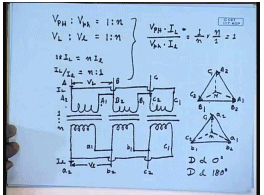So, the phase voltages and line voltages in this case are related by high voltage phase voltage is to low voltage side phase voltage are related as 1 is to n; same as the turns ratio. Also, the same relation holds; V line of the high voltage side and V line of the low voltage side are also related by 1 is to n. How about the currents? Let us say the line current on the high voltage side are I capital L and line currents on the low voltage side are I small l. Then if we assume each of this transformer to be ideal; that is, the net mmf is required to establish the flux is 0; then we can write 1 into I L since for this star connection, winding current and line current are same; equal to n into I small l. Therefore I L by I small l is also equal to n is to 1.

Hence, we have for this connection V phase into I phase; which is same as I line divided by V phase of the low voltage side into line current of the low voltage side equal to 1 by n into n by 1 equal to 1. Or, single phase KVA of the both sides are same. Hence, the 3 phase KVA of both sides are also same. Therefore, as we saw that, if the transformers are ideal; as in the case of a single phase transformer, the KVA of the two sides of a 3 phase transformer are also same. This connection is called the star-star connection or Y, Y connection. And since the phase angle between the line to neutral voltage of both sides are 0; it is also called star-star, 0 degree connection.

Obviously, on the secondary side, had we connected a 2, b 2 and c 2 together to form the neutral point and obtain the supply from the one terminal, then the phase and line voltages should have been in phase opposition to the primary side. These would have been V a 1 a 2; these would have been V b 1 b 2; and this would have been V c 1 c 2; in which case, the connection would have still been YY. But, now the phase difference between the primary side and the secondary side or the high voltage side and the low voltage side would have been 180 degree. And hence, this would have been called YY 180 degree connection. Just as the transformer on both sides can be connected in star, they can also be connected in delta.

Let us see how the delta connection can be done. As usual, the supply is given to the two terminals. And then we connect A 1 with B 2, B 1 with C 2, and C 1 with A 2. Supply is given to A, B, C. So, taking the voltage V B C as the reference phase, now B terminal is connected to B 2 and C terminals is connected to C 2; which in turn is connected to B 1. So, V B C is nothing but the voltage V B 2 B 1, which is shown here – B 2 B 1. And then terminal A 1 is connected to B 2 and A 2 is connected to C 1. This is voltage A 1 A 2 and this is voltage C 1 C 2. On the secondary side, these are the terminals a 2, b 2, c 2; and these are the terminals a 1, b 1, c 1. Similar connection is made; results in a similar phaser diagram. Please note here also the phase angle between line to neutral is 0. Therefore, this connection can be called delta-delta 0 degree.

Had we reversed the connection on the secondary side; that is, instead of connecting a 1 to b 2, we had connected b 1 to a 2, c 1 to b 2 and taken the supplies from a 1 b 1 c 1 instead of a 2 b 2 c 2; then this phaser diagram would have been reversed and we would have got D d 180 degree connection. In either case, let us see what will be the voltage and line conversion ratio. Again, we will assume the turns ratio to be 1 is to n and the line voltage on the high voltage side is V L, line current is I L. On the low voltage side, the line voltage we will take to be V l and the line current to be I l.

(Refer Slide Time: 40:55)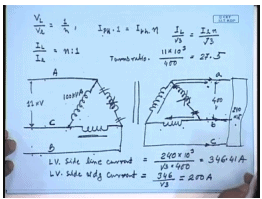Here again we find that here for delta connection, the winding voltage is same as the line voltage. Therefore, we can write V L by V l equal to 1 by n. But, winding current is now root 1 by root 3 times the line current, which is at a phase shift of 30 degree compared to the line current. This is phase current. But, these two phase currents are also co-phaser. And we have I phase into – on the high voltage side, into 1 equal to I phase on the low voltage side into n. But, I phase is I L by root 3 equal to – on the low voltage side, this is I small l into n by root 2. Here again we have I L by I small l equal to n is to 1. Hence, the relations for the star-star connection and the delta-delta connection between the phase voltages, line voltages and phase currents are same.

However, this star-star, delta-delta connection has one advantage. Let us assume that, we have a delta-delta connected transformer. Sometimes in order to indicate delta connection, the windings are placed like this although physically, in a transformer, it will not form a triangle; it will be rather what we have shown in this diagram. But, this is just a schematic representation. Suppose this was being supplied from a balanced 3 phase 3 wire supply and this was feeding a balanced 3 phase load; now, assume that, these deltadelta connected transformer is built with three single phase transformers. While operating, let us assume one of the transformers develops some fault and is consequently removed. Then the remaining transformer can still continue to supply some amount of load. When you remove these windings, you get the structure what is known as open delta or V-connected transformers. A V-connected transformer can continue to supply the load. That is one advantage of using three single phase transformers separately to form a 3 phase transformer rather than using a 3 phase transformer as a single unit. Sometimes this is important.

Let us see exactly how much KVA or power can be supplied with this open delta connected transformer or V-connected transformer through an example. Let us say the line voltage for this transformer was 11 KV; and on this side, it was 400 volts. And let us say each of these transformers are rated at 100 KVA. This is made of three single phase transformers rated at 100 KVA. And it was supplying a balanced star connected resistive load of 240 kilowatt. Assume the transformers to be ideal.

Therefore, if this load was drawing 240 kilowatt, then the input load was also 240 kilowatt. So, what was the line current? LV side line current was… This was 346.41 amperes. So, what was the LV side transformer winding current, which was LV side winding current? What was the transformer turns ratio from the given data? The transformer turns ratio was… On this side, the winding voltage is 11 KV; on this side, the winding voltage is 400 volts; the turns ratio was 27.5.

(Refer Slide Time: 48:28)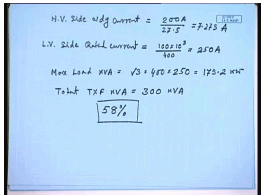So, what was the HV side winding current? HV side winding current was 200 ampere by turns ratio – 27.5; so 7.273 ampere. This was when it was operating as a 3 phase transformer. Now, let us say one of the transformers develops fault and is removed from the circuit. So, the transformer becomes a V-connected transformer or open delta connected transformer. The question is how much power I can still continue to supply to the load? The constant of course is that, the transformer winding current should not exceed its rating while being used as a V-connected transformer. So, ((Refer Time: 49:57)) what is the transformer winding rated current? We have mentioned that, each of the transformers is rated at 100 KVA. Therefore, the LV side rated current is transformer winding rated current is 100 KVA divided by 400 volt equal to 250 amperes.

So, when you are supplying this transformer with an open delta connection, the LV side winding current should not exceed 250 amperes. However, it is easy to see with open V connection, the line current on the LV side is same as the phase current, because this winding is not there; this line current flows like this and comes back through this; similarly, for the HV winding.

Therefore, the line current during open delta or V connection should not exceed 250 amperes. What about the phase? It is easy to see that, even with open delta or V connection, the line voltages will be balanced. This is so because the supply voltage is balanced; hence, the voltage across the remaining two transformers do not change. So, let us say if this was A, this was C, this was B; then this voltage still remains BC, this voltage still remains CA; therefore, this is still BC and CA. And hence, this voltage is ab. So, this low voltage side or LV side voltages still forms a balanced 3 phase system even during open delta connection. So, if this supply is balanced with open delta transformer, the LV side line voltages are balanced.

Now, if this balanced line voltage supplies a balanced 3 phase load; so the line currents will also be balanced. Hence, with open delta connection, we have seen that, that is, transformer will be able to supply a balanced 250 amperes of current. Hence, the total power that it will be able to supply is maximum load in kilowatt; or, load KVA should be equal to root 3 into 400 into 250 amperes; which is about 173.2 kilowatt. Please note that, the total rating of these transformers are 300 kilowatt; total transformer KVA is 300 KVA. Hence, with open delta, we can supply approximately 58 percent of the total transformer KVA. Please note that, we have removed one transformer. So, it was expected that, we should be able to supply about two-third or 67 percent of the total KVA; but that is not the case. We can supply only 58 percent of the remaining KVA of the total installed transformer KVA.Thank you.

Offer running on EduRev: Apply code STAYHOME200 to get INR 200 off on our premium plan EduRev Infinity!

## Electrical Machines for Electrical Engg.

22 videos|41 docs|26 tests

,

,

,

,

,

,

,

,

,

,

,

,

,

,

,

,

,

,

,

,

,

;